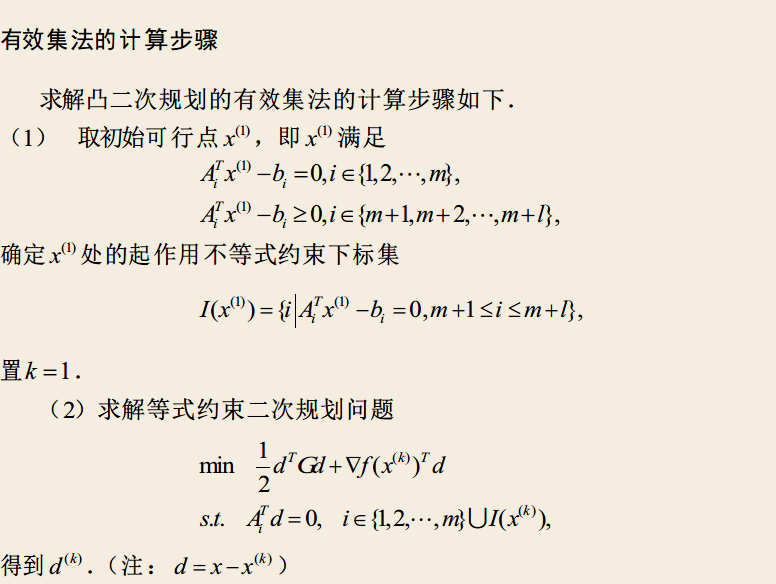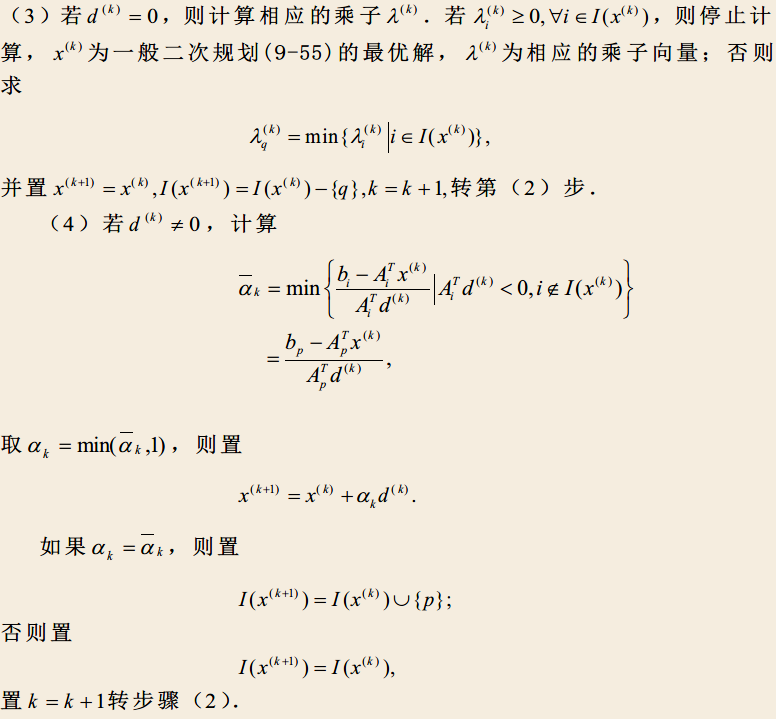$$min\;f(x)\\ s.t\quad c(x) \ge 0\\$$

• 目标函数：就是$f(x)$
• 决策变量：目标函数$f(x)$中的所有变量
• 约束条件：$c(x)$中包含的所有的等式和不等式
• 可行域：约束条件在空间围成的区域
• 可行解：可行域中的每个点都是原问题的一个可行点
• 最优解：能够使目标函数达到最大或最小的可行解
• 凸集：集合的一种，用于描述可行域，满足以下性质

令 $K$ 为集合，$\forall x_1,x_2 \in K$,若 $\alpha x_1 + (1-\alpha)x_2 \in K$,其中$\alpha \in [0,1])$,则 $K$ 为凸集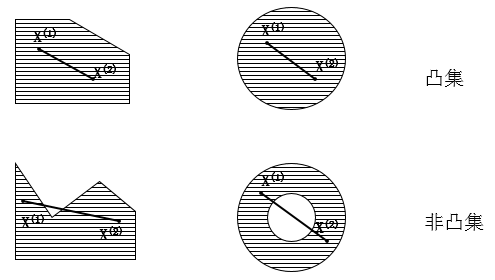## 线性规划

### 数学模型与基本概念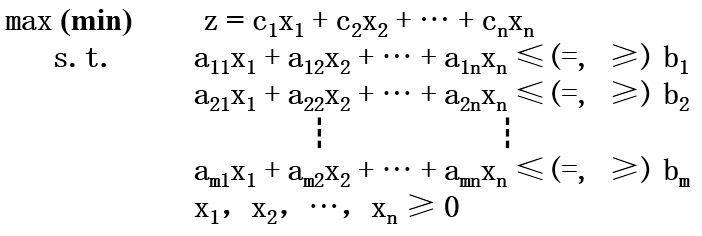1）目标函数求最小值
2）约束条件全为等式
3）所有的$x$有 $x \ge 0$

1）对于约束条件为 $\le$ 的情况，在 $\le$ 左边添加一个松弛变量(非负)。
2）对于约束条件为 $\ge$ 的情况，在 $\ge$ 左边减去一个剩余变量(非负)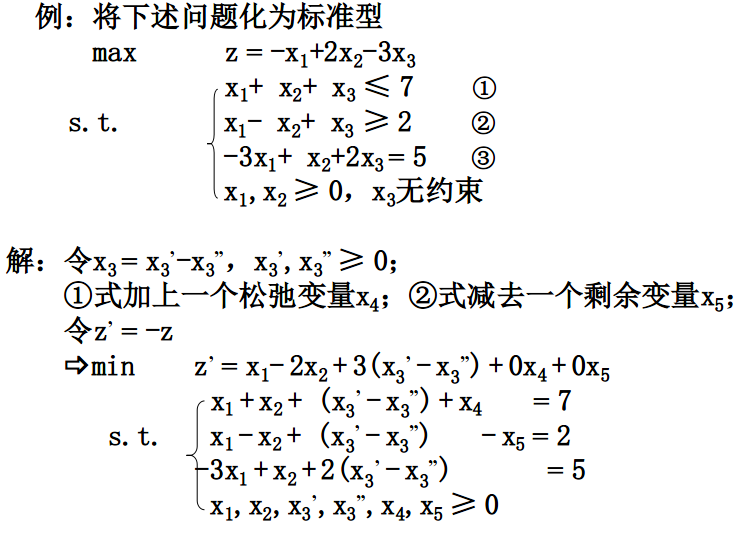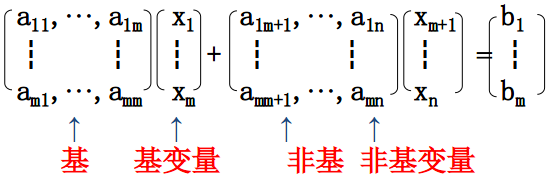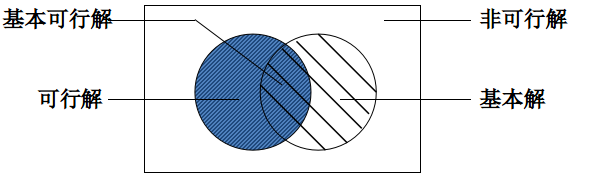### 单纯形法

1) 确定初始基本可行解
2) 判别当前基本可行解是否是最优解
3) 从一个基本可行解转换到相邻且改善了的基本可行解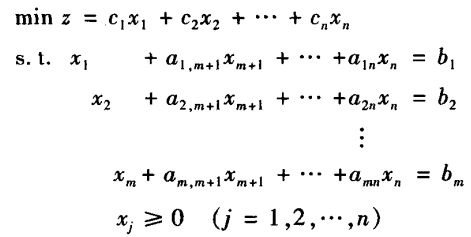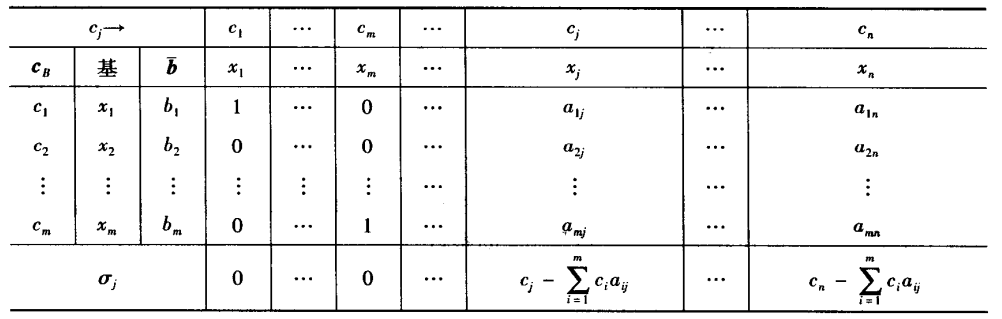（1）确定初始基本可行解

（2）判别当前基本可行解是否是最优解

（3）从一个基本可行解转换到相邻且改善了的基本可行解

$$\min_{1 \le i \le m} \lbrace \frac{b_i}{a_{ik}} | a_{ik} > 0 \rbrace$$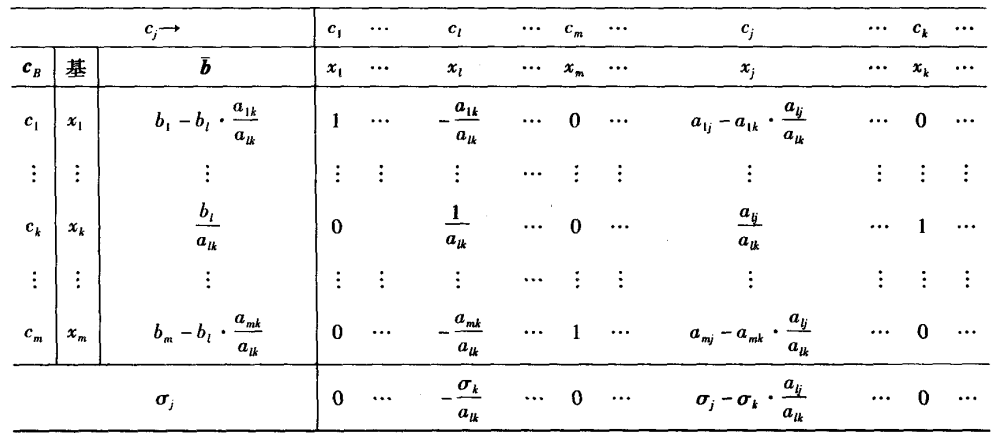（4）重复步骤（2）和（3），直到找到最优解

### 对偶理论

$$min\;z = c^Tx \quad \\ s.t.\,Ax \ge b(x \ge 0)\tag{1}$$

$$max\;w = b^Ty \quad \\ s.t.\,A^Ty \le c(y \ge 0)\tag{2}$$

1. max 问题第 i 个约束取“≥”，则min问题第 i 个变量 ≤ 0
2. min 问题第 i 个约束取“≤”，则max问题第 i 个变量 ≤ 0
3. 原问题第 i 个约束取等式，对偶问题第 i 个变量无约束
4. max 问题第 j 个变量 ≤ 0 ,则min问题第j个约束取“≤”
5. min 问题第 j 个变量 ≤ 0 ，则max问题第j个约束取“≥”
6. 原问题第j个变量无约束，对偶问题第j个约束取等式

max 问题任一可行解的目标值为min问题目标值的一个下界；
min 问题任一可行解的目标值为max问题目标值的一个上界

### 灵敏度分析

$$min\;z = c^Tx \quad \\ s.t.\,Ax \ge b(x \ge 0)\tag{1}$$

$c$ 的变化可分为两种：$c$为非基变量的价值系数和c为基变量的价值系数
1.非基变量价值系数 $c_k$的变化

2.基变量价值系数 $c_b$的变化

b变化的时候需要保证 $\overline{b} = B^{-1}(b+\Delta b) \ge 0$,否则需要将$\overline{b}$ 作为新的b的值代入到原来的单纯形表中，让后通过对偶单纯形法进行求解。对偶单纯形法的步骤与原始单纯形法的步骤非常相似，只是选择出基变量和入基变量的方法不同。出基变量选择为 $b_k = min\;\lbrace b_i,i=1,2,..m\rbrace$所对应的 $x_k$,入基变量选择为下面公式对应的$x_l$,

$$\frac{\sigma_l}{a_{kl}} = max\;[\frac{\sigma_j}{a_{kj}}|a_{kj} \lt 0,j=1,2,…n]$$

## 非线性规划

### 数学模型与基本概念

$$min f(x)\\ s.t\quad h_i(x) = 0(i=1,2,…,m)\\ \quad\quad g_j(x) \ge 0(j=1,2,…,l)\\$$

#### 梯度与海塞矩阵

$$\nabla^2 f(x) = \begin{pmatrix} \frac{\partial^2 f(x)}{\partial^2 x_1} & \frac{\partial^2 f(x)}{\partial x_1\partial x_2} & \cdots & \frac{\partial^2 f(x)}{\partial x_1\partial x_n}\\ \frac{\partial^2 f(x)}{\partial x_2\partial x_1} & \frac{\partial^2 f(x)}{\partial^2 x_2} & \cdots & \frac{\partial^2 f(x)}{\partial x_2\partial x_n}\\ \vdots & \vdots & \ddots & \vdots \\ \frac{\partial^2 f(x)}{\partial x_n\partial x_1}& \frac{\partial^2 f(x)}{\partial x_n\partial x_2} & \cdots & \frac{\partial^2 f(x)}{\partial^2 x_n}\\ \end{pmatrix}$$

#### 凸函数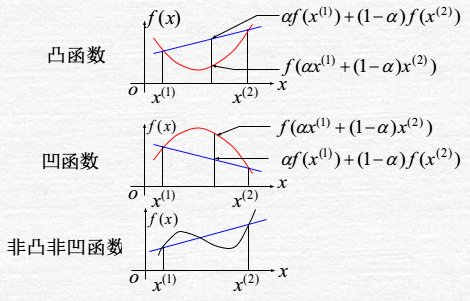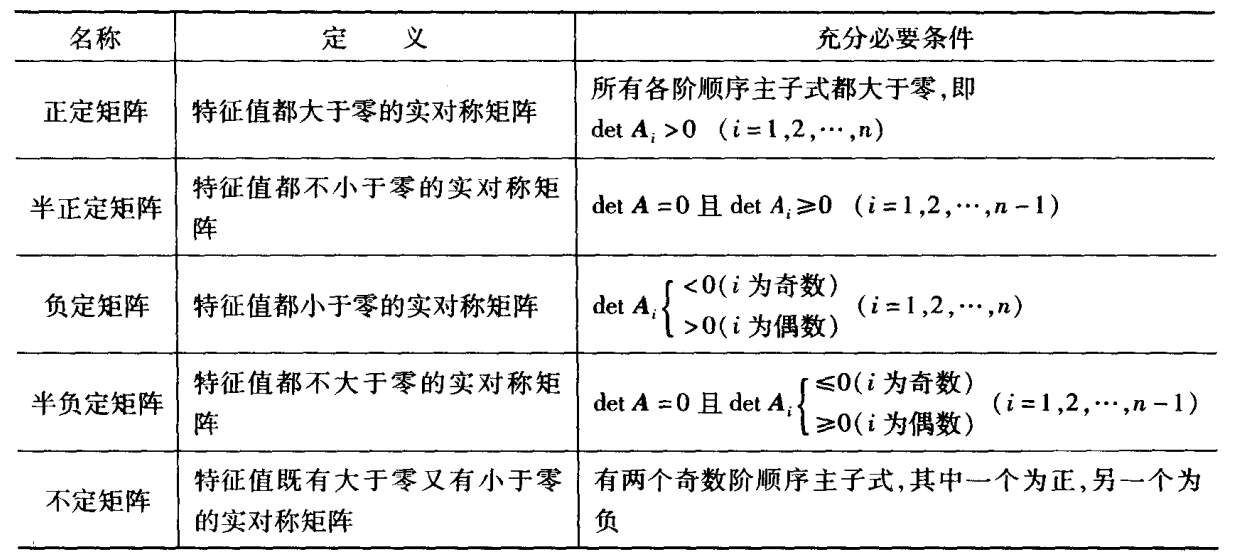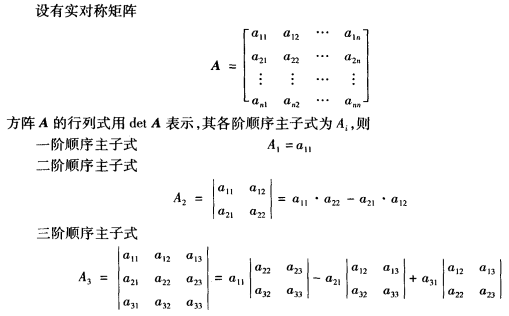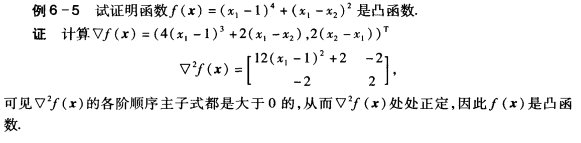#### 凸规划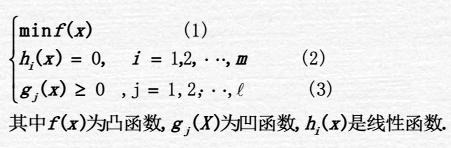### 一维搜索

#### 单谷函数

1)若$f(x_1)≥f(x_2),\overline x\in [x_1,b]$
2)若$f(x_1) < f(x_2),\overline x \in [a,x2]$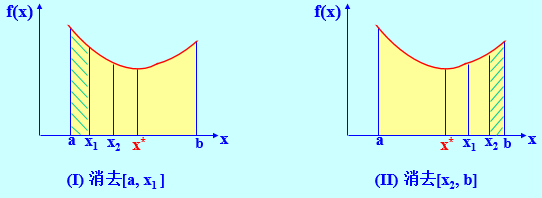#### 外推内插法

1.选定初始点a 和步长h;

2.计算并比较$f(a)$和$f(a+h)$；有前进和后退两种情况：
1)前进算法：若$f(a) \ge f(a+h)$则步长加倍，计算$f(a+3h)$。若$f(a+h) \le f(a+3h)$，$a_1=a, a_2=a+3h$, 停止运算；否则将步长加倍，并重复上述运算。
2)后退算法：若$f(a) \lt f(a+h)$则步长改为 $-h$，计算$f(a-h)$。若$f(a-h) \ge f(a)$，$a_1=a-h, a_2=a+h$, 停止运算；否则将步长加倍，继续后退。

3.得到的满足“中间小两头大”的三点已经可以作为单谷区间，但是当这个区间太大的时候，也可以进行缩短，缩短的方法如下：

#### 三点二次插值法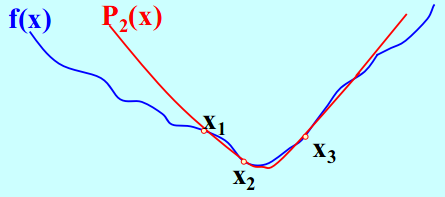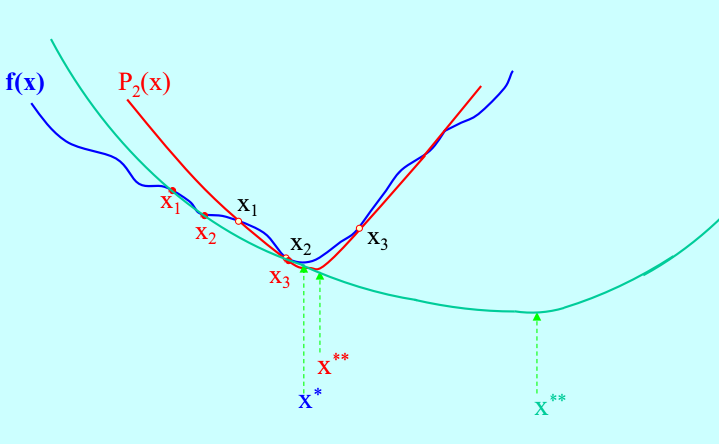#### 牛顿法

$$f(x) \approx \varphi(x) = f(x_k)+f^{‘}(x_k)(x-x_k)+\frac{1}{2}f^{“}(x_k)(x-x_k)^2$$

$$x_{k+1} = x_k - \frac{f^{‘}(x_k)}{f^{“}(x_k)}$$

### 无约束非线性规划

1. 直接法：求解过程中只用到目标函数值，无须计算导数。如变量轮换(坐标轮换)，模式搜索等。
2. 解析法：用函数的解析性（一阶、二阶导数），即在计算过程中需要计算目标函数的导数。如：梯度法、共扼梯度法、牛顿法

#### 直接法

##### 坐标(变量)轮换法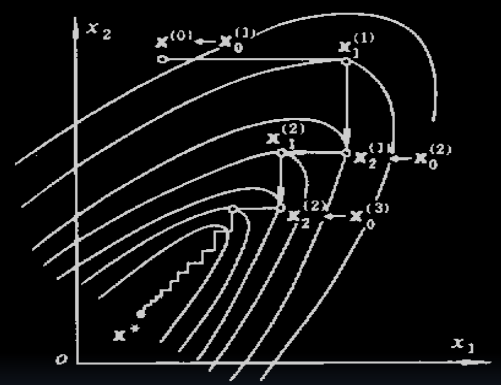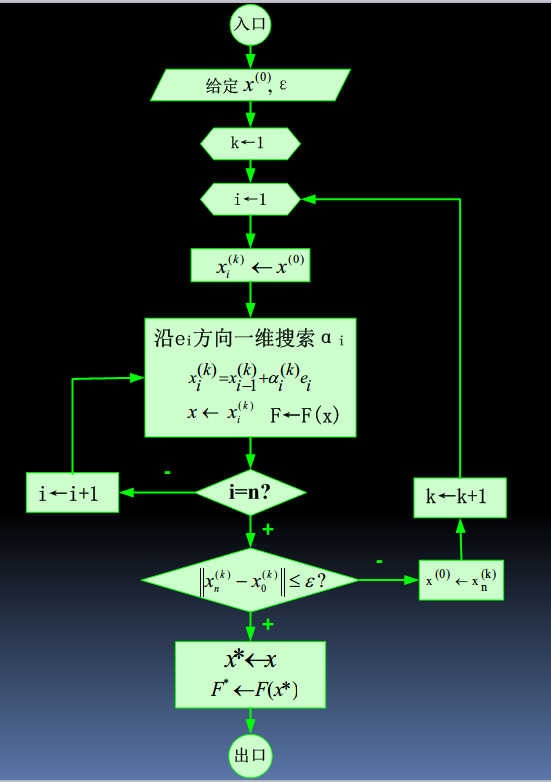##### 模式搜索法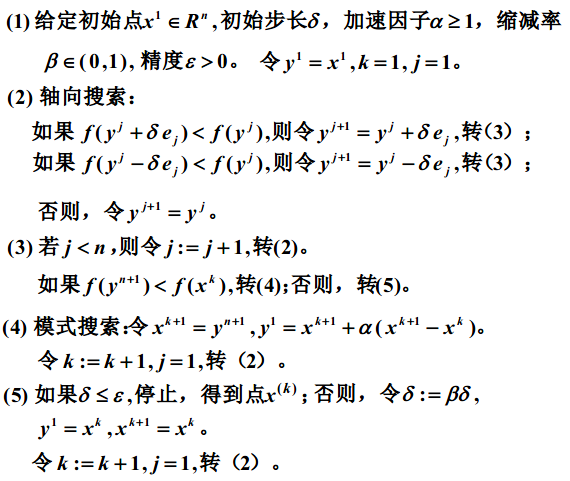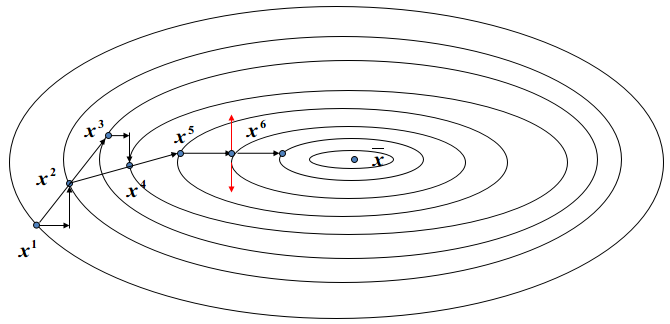##### 可变单纯形法

Lagarias(1998)研究了可变单纯形法求解低维函数时的收敛特性，但结论难以推广到高维问题，也即单一可变单纯形法难以保证对高维复杂函数具有较好的优化效果。

#### 解析法

##### 最速下降法

$$x^{(k+1)} = x^{(k)} + \alpha^{(k)}d^{(k)}$$

$$f(x^{(k)} + \alpha^{(k)}d^{(k)}) =min\; f(x^{(k)} + \alpha d^{(k)}) = min\;\varphi(\alpha)$$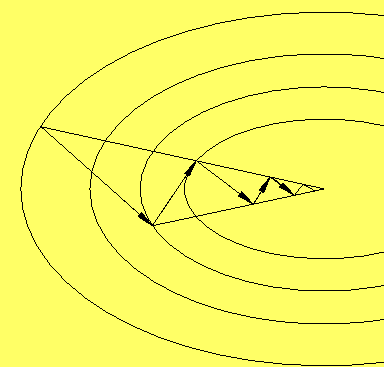##### 牛顿法

###### 原始牛顿法

$$f(x) \approx \varphi(x) = f(x_k)+f^{‘}(x_k)(x-x_k)+\frac{1}{2}f^{‘’}(x_k)(x-x_k)^2$$

$$f(x) \approx \varphi(x) = f(x^{(k)})+\nabla f(x^{(k)})^T\Delta x+\frac{1}{2}\Delta x^TH_k\Delta x$$

###### 阻尼牛顿法

$x^{(k+1)} = x^{(k)}-H_k^{-1}\nabla f(x^{(k)})$

$x^{(k+1)} = x^{(k)}-\alpha^{(k)}H_k^{-1}\nabla f(x^{(k)})$

1）对目标函数要求苛刻，要求函数具有连续的一、二阶导数；为保证函数的稳定下降，海赛矩阵必须正定；为求逆阵要求海赛矩阵非奇异。
2）计算复杂且计算量大，存储量大

###### 拟牛顿法(变尺度法)

$x^{(k+1)} = x^{(k)}-\alpha^{(k)}A_k\nabla f(x^{(k)})$

##### 共轭梯度法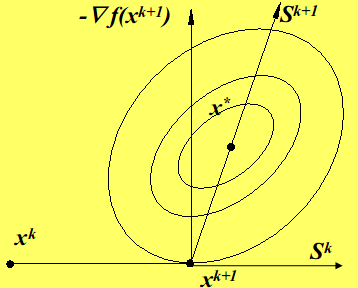1）以 $x^{(k+1)}$ 点出发的搜索方向 $S^{k+1}$ 是$-\nabla f(x^{(k+1)})$ 与 $S^k$ 的线性组合。即
$$S^{k+1} = -\nabla f(x^{(k+1)}) + \beta_kS^k$$

$$\beta_k = (\frac{||\nabla f(x^{(k+1)})||}{||\nabla f(x^{(k)})||})^2$$

2）$[S^{k+1}]^TGS^k=0$

$x^{(k+1)} = x^{(k)}+\alpha^{(k)}S^{(k)}$

$||\nabla f(x^{(k+1)})|| \lt \epsilon$ 是否成立即可

#### 方法对比

1、可靠性。即在合理的精度要求下，在一定允许时间内能解出各种不同类型问题的成功率。能够解出的问题越多，则算法的可靠性越好
2、有效性。即算法的解题效率。它有两个衡量标准。其一是对同一题目，在相同精度和初始条件下，比较机时多少。其二是在相同精度下，计算同一题目所需要的函数的计算次数。
3、简便性。一方面指实现该算法的准备工作量的大小。另一方面指算法占用存储单元的数量。

1、一般而言，对于维数较低或者很难求得导数的目标函数，使用坐标轮换法较合适。
2、对于二次性较强的目标函数，使用牛顿法效果好。
3、对于一阶偏导数易求的目标函数，使用梯度法可使程序编制简单，但精度不宜过高
4、综合而言，共轭梯度法和DFP法具有较好的性能。

### 约束非线性规划

$$min\;f(x)\\ s.t\quad h_i(x) = 0(i=1,2,…,m)\\ \quad\quad g_j(x) \ge 0(j=1,2,…,l)\\$$

$I(x) = \lbrace j|g_j(x)=0, 1 \le j \le l \rbrace$

#### KT条件

KT条件是非线性规划领域中最重要的理论成果之一，其重要的意义在于它是确定某点是最优点的一阶必要条件，只要是最优点就必须满足这个条件。但一般来说它不是充分条件，即满足KT条件的点并不一定是最优点。但是对于凸规划，KT条件是最优点存在的充要条件。也就是说在凸规划中通过KT条件可以找到最优解。

$$min\;f(x)\\ s.t\quad h_i(x) = 0(i=1,2,…,m)\\ \quad\quad g_j(x) \ge 0(j=1,2,…,l)\\$$

$$\nabla f(x) - \sum_{i=1}^mr_i\nabla h_i(x) - \sum_{j=1}^l\lambda_j\nabla g_j(x) = 0\\ \lambda_j g_j(x) = 0\quad (j=1,2,3…l)\\ \lambda_j \ge 0 \quad (j=1,2,3…l)\\$$

$f(x) - \sum_{i=1}^mr_i h_i(x) - \sum_{j=1}^l\lambda_j g_j(x)$

$(r_1,r_2,…r_m)$ 和 $(\lambda_1,\lambda_2,…\lambda_l)$

#### 罚函数法

##### 外点法

$$min\;f(x)\\ s.t\quad h_i(x) = 0(i=1,2,…,m)\\$$

$$min\;p(x,M) = f(x) + M\sum_i^m [h_i(x)]^2$$

$$min\;f(x)\\ s.t\quad g_j(x) \ge 0(j=1,2,…,l)\\$$

$$p(x,M) = f(x) + M\sum_j^l [min(0, g_j(x))]^2$$

$$p(x,M) = f(x) + M( \sum_i^m [h_i(x)]^2 + \sum_j^l [min(0, g_j(x))]^2)$$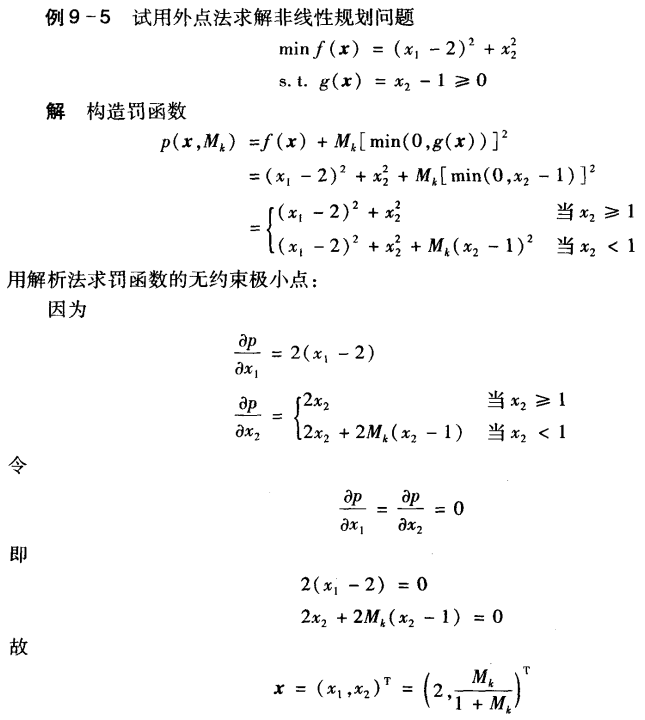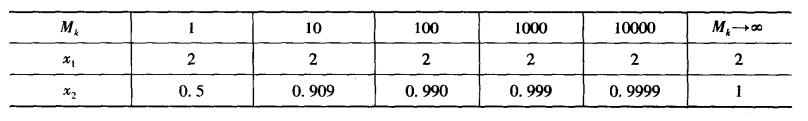##### 内点法

$$min\;f(x)\\ s.t\quad g_j(x) \ge 0(j=1,2,…,l)\\$$

$H = \lbrace g_j(x) > 0 |j=1,2,…,l\rbrace$

$$p(x,r_k) = f(x) + r_k \sum_j^l \frac{1}{g_j(x)}$$

$$p(x,r_k) = f(x) - r_k \sum_j^l ln(g_j(x))$$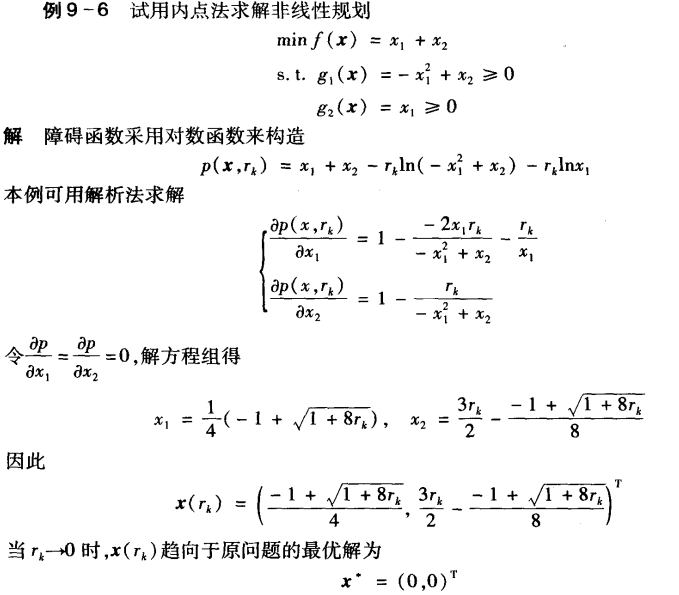##### 乘子法

$$min\;f(x)\\ s.t\quad h_i(x) = 0(i=1,2,…,m)\\$$

$$\varphi (x,\lambda, M) = f(x) - \sum_{i=0}^m \lambda_i h_i(x) + \frac{M}{2} \sum_{i=1}^m [h_i(x)]^2$$

$$\overrightarrow {\lambda^{(k+1)}} = \overrightarrow {\lambda^{(k)}} - Mh_i(x^{(k)})$$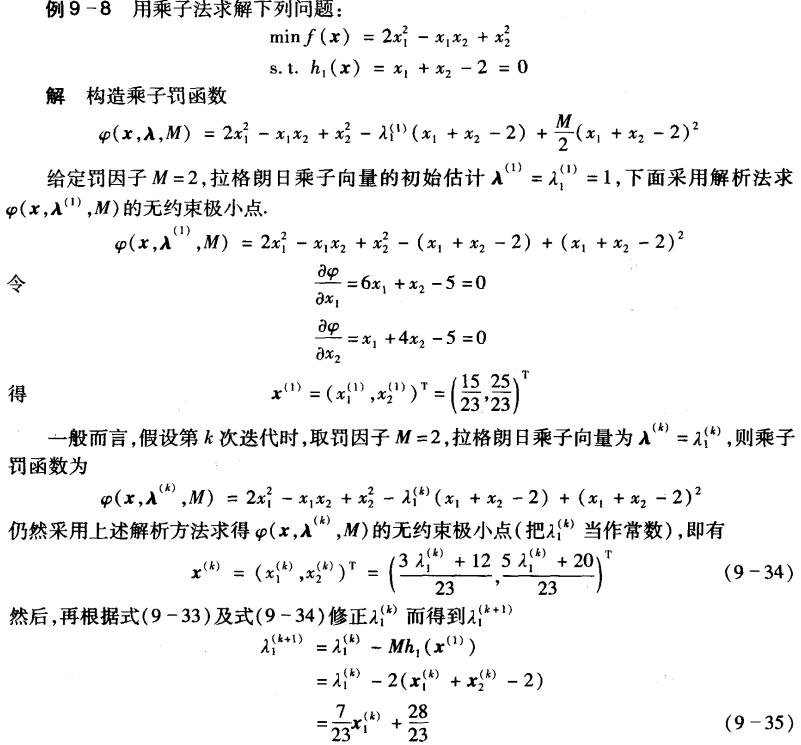#### 二次规划

$$min\;f(x)\\ s.t\quad h_i(x) = 0(i=1,2,…,m)\\ \quad\quad g_j(x) \ge 0(j=1,2,…,l)\\$$

$$min\;f(x) = \frac{1}{2}x^TGx + r^Tx\\ s.t\quad A_i^Tx - b_i = 0(i=1,2,…,m)\\ \quad\quad\quad A_i^Tx-b_i \ge 0(i=m+1,…,m+l)\\$$

$$G\overline x + r - \sum_{i=1}^{m+l}\lambda_iA=0\\ \lambda_i (A_i^Tx-b_i) = 0(i=m+1,…,m+l)\\ \lambda_i \ge 0(i=m+1,…,m+l)$$

$I(x) = \lbrace i| A_i^Tx =b_i, m+1 \le i \le m+l\rbrace$

$E = \lbrace 1,2,…m\rbrace \bigcup I(x)$

$$min\;f(x) = \frac{1}{2}x^TGx + r^Tx\\ s.t\quad A_i^Tx = b_i (i \in E)\\$$